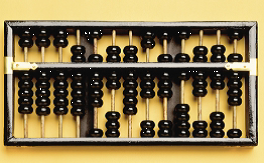Computer History:

The tool known as “ABACUS” was the age-old tool used by man to count and calculate was designed and developed by Chinese (Asia Minor) nearly 5000 years ago. The abacus may be considered the first computer. This device allowed its users to make computations using a system of sliding beads arranged on a rack. Early shopkeepers used the abacus to keep up with transactions. The use of pencil and paper spread, the abacus lost its importance. Nearly twelve centuries past before the next important advance in computing devices emerged.Abacus
In 1642, Blaise Pascal, the 18-year-old son of a French tax collector, invented what he called a numerical wheel calculator to help his father with his duties. The Pascaline, a brass rectangular box, used eight movable dials to add sums up to eight figures long. Pascal's device used a base of ten to achieve this. The disadvantage to the Pascaline, of course, was its limitation to addition. In 1694, Gottfried Wilhem von Leibniza a German mathematician and philosopher improved the Pascaline by creating a machine that could also multiply. Like its predecessor, Leibniz's mechanical multiplier worked by a system of gears and dials.

It wasn't until 1820, however, that mechanical calculators gained widespread use. A Frenchman, Charles Xavier Thomas de Colmar, invented a machine that could perform the four basic mathematical functions. The arithmetic, presented a more systematic approach to computing because it could add, subtract, multiply and divide. With its enhanced versatility, the arithmetic was widely used up until World war.

In 1833 “Charles Babbage” an English scientist, designed Analytical Engine using tooth wheels so that it could perform all mathematical operations in a predetermined sequence using a set of operational instructions, so he is called as a “father of computers”.Charles Babbage (Father of Computer)
Lady Ada Lovelace is considered as the first lady Computer Programmer as she has developed the concept of writing systematic operational instructions for the Analytical Engine.Lady Ada Lovelace ( 1815 --  1852 )

In 1850 George Boole an English mathematician proposed logic theory of using the binary (two) number system. This had only two numbers 0 and 1. In this procedures all the quantities are represented in terms of 0 and 1 for example 9 is represented as 00001001.George Boole ( 1815 -- 1864 )
Bool proposed a logic popularly known as Boolean Algebra, Computer processors are designed on this system of logic.